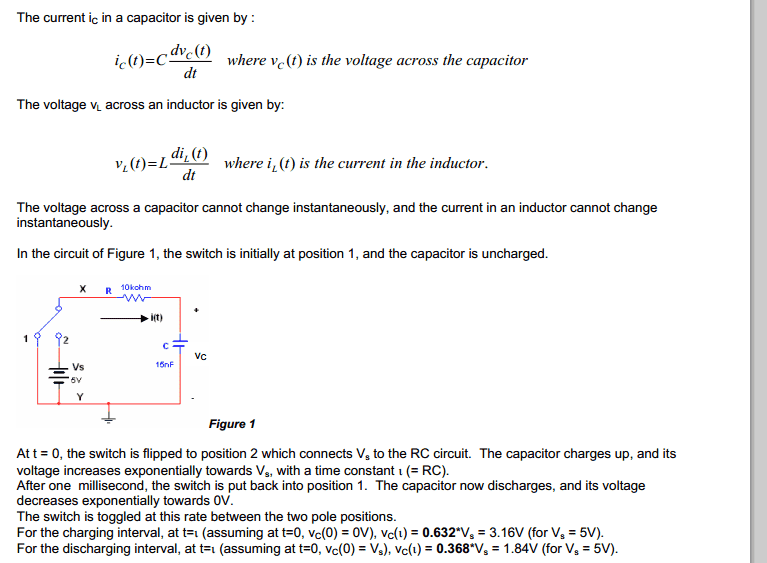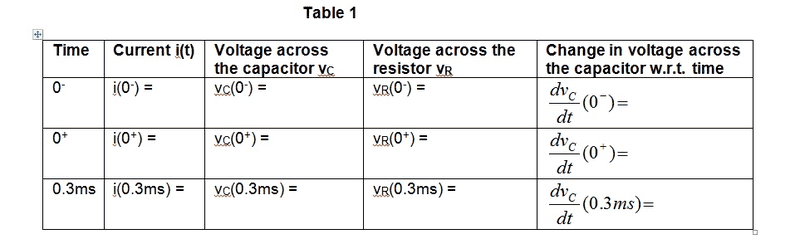# First order RC Circuit Table problem

## Homework Statement

Hi I got this problem and have to fill out this table.## Homework Equations

node analysis
time constant = RC
ic(t)= C dVc(t)/dt

## The Attempt at a Solution

I got few answers which i worked it out.

i (0-) = 0

Vc(0-) = 1.84

Vr(0-) = i (0-) * 10000

i (0+) = 0

Vc(0+) = 3.16

Vr(0+) = i (0-) * 10000

Am i right so far?
I dont know rest of them. the last column i know i have to use node analysis for that.

gneill
Mentor
The problem description mentions two separate time intervals, a charging interval and a discharging interval. You haven't stated which interval is in play for the determination of the table values.

I understand but thats what it said... complete table 1.

The problem description mentions two separate time intervals, a charging interval and a discharging interval. You haven't stated which interval is in play for the determination of the table values.

I understand but thats what it said... complete table 1.

gneill
Mentor
I understand but thats what it said... complete table 1.

Okay, so pick one of the intervals and state which one you've chosen (somehow I suspect that they want you to fill out the table twice, once for each interval...).

Hint: Before you make assumptions about Vc(0-) be sure to compare the time constant of the circuit to the given switching interval.